# 1.5: 小数

•• OpenStax
• OpenStax
$$\newcommand{\vecs}{\overset { \rightharpoonup} {\mathbf{#1}} }$$ $$\newcommand{\vecd}{\overset{-\!-\!\rightharpoonup}{\vphantom{a}\smash {#1}}}$$$$\newcommand{\id}{\mathrm{id}}$$ $$\newcommand{\Span}{\mathrm{span}}$$ $$\newcommand{\kernel}{\mathrm{null}\,}$$ $$\newcommand{\range}{\mathrm{range}\,}$$ $$\newcommand{\RealPart}{\mathrm{Re}}$$ $$\newcommand{\ImaginaryPart}{\mathrm{Im}}$$ $$\newcommand{\Argument}{\mathrm{Arg}}$$ $$\newcommand{\norm}{\| #1 \|}$$ $$\newcommand{\inner}{\langle #1, #2 \rangle}$$ $$\newcommand{\Span}{\mathrm{span}}$$ $$\newcommand{\id}{\mathrm{id}}$$ $$\newcommand{\Span}{\mathrm{span}}$$ $$\newcommand{\kernel}{\mathrm{null}\,}$$ $$\newcommand{\range}{\mathrm{range}\,}$$ $$\newcommand{\RealPart}{\mathrm{Re}}$$ $$\newcommand{\ImaginaryPart}{\mathrm{Im}}$$ $$\newcommand{\Argument}{\mathrm{Arg}}$$ $$\newcommand{\norm}{\| #1 \|}$$ $$\newcommand{\inner}{\langle #1, #2 \rangle}$$ $$\newcommand{\Span}{\mathrm{span}}$$$$\newcommand{\AA}{\unicode[.8,0]{x212B}}$$

##### 学习目标

• 四舍五入小数
• 加上和减去小数
• 乘以和除以小数
• 转换小数、分数和百分比
• 使用平方根简化表达式
• 识别整数、有理数、非理数和实数
• 在数字行上找到分数和小数

## 四舍五进制小数

$\begin{array}{rcll} 0.1 & = & \dfrac{1}{10} & \text{is “one tenth”} \\ 0.01 & = & \dfrac{1}{100} & \text{is “one hundredth”} \\ 0.001 & = & \dfrac{1}{1000} & \text{is “one thousandth”} \\ 0.0001 & = & \dfrac{1}{10,000} & \text{is “one ten-thousandth”} \end{array}$

##### 四舍五入小数。
1. 找到给定的位值并用箭头标记。
2. 为位值右边的数字加下划线。
3. 带下划线的数字是否大于或等于 5？
• 是：在给定位数值的数字上加 1。
• 否：请更改给定位数值中的数字
4. 重写数字，删除四舍五入数字右侧的所有数字。
##### 示例$$\PageIndex{1}$$

ⓐ 到最接近的百分之一

 用箭头找到第100个位置。为给定位数右边的数字加下划线。因为 9 大于或等于 5，所以将 1 与 7 相加。重写数字，删除四舍五入数字右侧的所有数字。请注意，删除的数字并未替换为零。ⓑ 到最接近的十分之一

 用箭头找到第十个位置。为给定位数右边的数字加下划线。由于 7 大于或等于 5，因此将 1 与 3 相加。重写数字，删除四舍五入数字右侧的所有数字。请注意，删除的数字并未替换为零。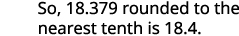ⓒ 到最接近的整数

 用箭头找到那个地方。为给定位数右边的数字加下划线。由于 3 不大于或等于 5，因此请勿将 1 与 8 相加。重写数字，删除四舍五入数字右侧的所有数字。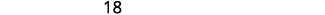##### 示例$$\PageIndex{2}$$

$$6.58$$$$6.6$$$$7$$

##### 示例$$\PageIndex{3}$$

$$15.218$$$$15.22$$

$$15.2$$

## 加上和减去小数

##### 加上或减去小数。
1. 确定总和或差值的符号。
2. 写下数字，使小数点垂直排列。
3. 根据需要使用零作为占位符。
4. 将数字相加或减去，就好像它们是整数一样。 然后将答案中的
小数点放在给定数字的小数点下方。
5. 用相应的符号写下总和或差值。
##### 示例$$\PageIndex{4}$$

$$\begin{array}{ll} \text{} & −23.5−41.38 \\ \\ \\ {\text{The difference will be negative. To subtract, we add the} \\ \text{numerals. Write the numbers so the decimal points line} \\ \text{up vertically.}} & { \; \; 23.5 \\ \underline{+41.38}} \\ \\ \\ { \text{Put 0 as a placeholder after the 5 in 23.5.} \\ \text{Remember, } \frac{5}{10}=\frac{50}{100} \text{ so } 0.5=0.50.} & { \; \; 23.50 \\ \underline{+41.38}} \\ \\ \\ {\text{Add the numbers as if they were whole numbers.} \\ \text{Then place the decimal point in the sum.}} & {\; \; 23.50 \\ \underline{+41.38} \\ \; \; 64.88 } \\ \\ \\ \text{ Write the result with the correct sign.} & 64.88−23.5−41.38=−64.88 \end{array}$$

$$\begin{array}{ll} \text{} & 14.65−20 \\ \\ \\ {\text{The difference will be negative. To subtract, we} \\ \text{subtract 14.65 from 20.}} \\ \\ \\ {\text{Write the numbers so the decimal points line up} \\ \text{vertically.}} & { \; \; 20 \\ \underline{−14.65}} \\ \\ \\ {\text{Remember, 20 is a whole number, so place the} \\ \text{decimal point after the 0.}} \\ \\ \\ \text{Put in zeros to the right as placeholders.} & { \; \; 20.00 \\ \underline{−14.65}} \\ \\ \\ \text{Subtract and place the decimal point in the answer.} & {\begin{array}{lcccc} {} & 9 & {} & 9 & {} \\ 1 & \cancel{10} & {} & \cancel{10} & 10 \\ 2 & 0 & . & 0 & 0 \\ −1 & 4 & . & 6 & 5 \end{array} \\ \text{______________________} \\ \begin{array}{lcccc} {\; \; \; \; \; \; \; \; \; } & 5 & . & 3 & 5 \end{array}} \\ \\ \\ \text{Write the result with the correct sign.} & 14.65−20=−5.35 \end{array}$$

##### 示例$$\PageIndex{5}$$

$$−16.49$$$$−0.42$$

##### 示例$$\PageIndex{6}$$

$$−23.593$$$$−12.58$$

## 乘以和除以小数

##### 乘以小数。
1. 确定产品的标志。
2. 以垂直格式书写，将右边的数字排成一列。 将数字相乘，就好像它们是整数一样，暂时忽略小数点。
3. 放置小数点。 乘积中的小数位数是因子中小数位数的总和。
4. 在产品上写上相应的标志。

 $$(−3.9)(4.075)$$ 迹象不同。 该产品 将是负面的。 该产品将是负面的。 以垂直格式书写，将右边的 数字对齐。乘。将因子中的 小数位数相加 (1 + 3)。 将小数点置于右边 4 位。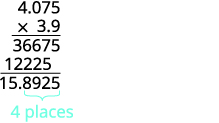迹象不同，所以产品是负面的。 $$(−3.9)(4.075)=−15.8925$$

$$−27.4815$$

##### 示例$$\PageIndex{9}$$

$$−87.6148$$

##### 将@@ 十进制乘以十的乘方。
1. 将小数点向右移动，其位
数与 10 的幂次数相同。
2. 根据需要在数字末尾添加零。
##### 示例$$\PageIndex{10}$$10 中有 1 个零，因此将小数点向右移动 1 位。100 中有 2 个零，因此将小数点向右移动 2 位。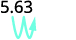1,000 中有 3 个零，因此将小数点向右移动 3 位。必须将零加到末尾。##### 示例$$\PageIndex{11}$$

ⓐ 25.8 ⓑ 258 ⓒ 2,580

##### 示例$$\PageIndex{12}$$

ⓐ 142 ⓑ 1,420 ⓒ 14,200##### 除以小数。
1. 确定商的符号。
2. 将小数点一直向右 “移动”，使除数成为整数。 将除数中的小数点 “移动” 到相同数量的位数——根据需要添加零。
3. 除以。 将小数点置于除息小数点上方的商数中。
4. 用适当的符号写出商。
##### 示例$$\PageIndex{13}$$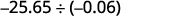迹象是一样的。 商是正数。 将小数点一直向右 “移动”，使除数成为整数。 将除数中的小数点 “移动” 相同的位数。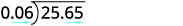除以。 将小数点置于除息小数点上方的商数中。用适当的符号写出商。##### 示例$$\PageIndex{14}$$

$$587.3$$

##### 示例$$\PageIndex{15}$$

$$34.25$$

## 转换小数、分数和百分比

$0.03=\dfrac{3}{100}$

##### 将小数转换为正确的分数，将分数转换为十进制。
1. 要将小数转换为正确的分数，请确定最后一个数字的位值。
2. 写下分数。
• 分子-小数点右边的 “数字”
• 分母-与最后一位数字对应的位值
3. 要将分数转换为十进制，请将分数的分子除以分数的分母。
##### 示例$$\PageIndex{16}$$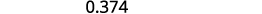确定最后一位数字的位值。写下 0.374 的分数：分子是 374。 分母为 1,000。简化分数。除去常见因素。ⓑ 由于分数条表示除法，因此我们首先将分数写$$\frac{5}{8}$$$$8\sqrt{5}$$。 现在分开。##### 示例$$\PageIndex{17}$$

$$\frac{117}{500}$$$$−0.875$$

##### 示例$$\PageIndex{18}$$

$$\frac{3}{125}$$$$−0.375$$##### 将@@ 百分比转换为十进制，将小数转换为百分比。
1. 要将百分比转换为小数，请在删除百分号后将小数点向左移动两位。
2. 要将小数转换为百分比，请将小数点向右移动两位，然后添加百分号。
##### 示例$$\PageIndex{19}$$

ⓐ 百分比到十进制：62%、135% 和 13.7%。

ⓑ 十进制到百分比：0.51、1.25 和 0.093。将小数点向左移动两位。将小数点向右移动两位。##### 示例$$\PageIndex{20}$$

ⓐ 百分比到十进制：9%、87% 和 3.9%。

ⓑ 十进制到百分比：0.17、1.75 和 0.0825。

ⓐ 0.09、0.87、0.039 ⓑ 17%、175%、8.25%

##### 示例$$\PageIndex{21}$$

ⓐ 百分比到十进制：3%、91% 和 8.3%。

ⓑ 十进制到百分比：0.41、2.25 和 0.0925。

ⓐ 0.03、0.91、0.083 ⓑ 41%、225%、9.25%

## 使用平方根简化表达式

##### 数字的平方

if$$n^2=m$$，那么 mn平方

$(−3)^2=9 \; \; \; \; \; \; \; \; \; (−8)^2=64 \; \; \; \; \; \; \; \; \; (−11)^2=121 \; \; \; \; \; \; \; \; \; (−15)^2=225$

##### 数字的平方根

if$$n^2=m$$，则 nm平方根

$$(−10)^2=100$$另请注意，所以 −10 也是 100 的平方根。 因此，10 和 −10 都是 100 的平方根。 因此，每个正数都有两个平方根——一个正数和一个负数。 激进符号$$\sqrt{m}$$，表示正平方根。 正平方根称为主平方根。 当我们使用激进符号时，这总是意味着我们想要主平方根。

##### 平方根表示法

$$\sqrt{m}$$读作 “mm 的平方根”。m 的平方根是正数$$\sqrt{m}$$，其平方为 m

##### 练习$$\PageIndex{22}$$

$$\begin{array}{ll} \text{} & \sqrt{25} \\ \text{Since }5^2=25 & 5 \end{array}$$

$$\begin{array}{ll} \text{} & \sqrt{121} \\ \text{Since }11^2=121 & 11 \end{array}$$

$$\begin{array}{ll} {} & −\sqrt{144} \\ \text{The negative is in front of} & −12 \\ \text{the radical sign.} \end{array}$$

ⓐ 6 ⓑ 13 ⓒ −15

ⓐ 4 ⓑ 14 ⓒ −10

## 识别整数、有理数、非理数和实数

$\begin{array}{ll} \text{Counting numbers} & 1,2,3,4,….. \\ \text{Whole numbers} & 0,1,2,3,4,…. \\ \text{Integers} & ….−3,−2,−1,0,1,2,3,…. \end{array}$

##### 有理数

$π=3.141592654...$

##### 理性还是非理性

• 重复或停止，该数字是一个有理数
• 不重复也不停止，这个数字是一个非理性的数字。

##### 实数

“实数” 一词对你来说似乎很奇怪吗？ 有没有不是 “真实” 的数字，如果有，它们可能是什么？ 我们可以简化$$−\sqrt{25}$$吗？ 有数字的正方形$$−25$$吗？

$()^2=−25?$

##### 练习$$\PageIndex{25}$$

ⓐ 记住，整数$$0,1,2,3,…,$$是 8 是唯一给出的整数。

ⓑ 整数是整数及其对立面（包括 0）。 所以整数 8 是一个整数，而 −7 与整数相反，所以它也是一个整数。 另外，请注意，64 是 8 的平方，所以$$−\sqrt{64}=−8$$。 所以整数是$$−7,8,$$$$−\sqrt{64}$$

ⓒ 由于所有整数都是有理的，那么$$−7,8,$$$$−\sqrt{64}$$都是有理的。 有理数还包括重复或停止的分数和小数，因此$$\frac{14}{5}$$$$5.9$$是有理的。 因此，有理数列表是$$−7,\frac{14}{5},8,5.9,$$$$−\sqrt{64}$$

ⓓ 请记住，5 不是一个完美的正方形，所以$$\sqrt{5}$$是不合理的。

ⓔ 列出的所有数字均为实数。

##### 练习$$\PageIndex{26}$$

ⓓ 非理性数字 ⓔ 实数。

$$4,\sqrt{49}$$$$−3,4,\sqrt{49}$$

$$−3,0.\overline{3},\frac{9}{5},4,\sqrt{49}$$$$−\sqrt{2}$$

$$−3,−\sqrt{2},0.\overline{3},\frac{9}{5},4,\sqrt{49}$$

##### 练习$$\PageIndex{27}$$

$$6,\sqrt{121}$$

$$−\sqrt{25},−1,6,\sqrt{121}$$

$$−\sqrt{25},−\frac{3}{8},−1,6,\sqrt{121}$$

$$2.041975...$$

$$−\sqrt{25},−\frac{3}{8},−1,6,\sqrt{121},2.041975...$$

## 在数字行上找到分数和小数

$\dfrac{7}{4}=1\dfrac{3}{4} \; \; \; \; \; \; \; \; \; −\dfrac{9}{2}=−4\dfrac{1}{2} \; \; \; \; \; \; \; \; \; \dfrac{8}{3}=2\dfrac{2}{3}$##### 练习$$\PageIndex{28}$$

$$\frac{3}{4}$$首先找到正确的分数。 分数介$$\frac{3}{4}$$于 0 和 1 之间。 将 0 和 1 之间的距离分成四个相等的部分，然后我们绘制图$$\frac{3}{4}$$。 同样的情节$$−\frac{1}{4}$$##### 练习$$\PageIndex{29}$$##### 练习$$\PageIndex{30}$$##### 练习$$\PageIndex{31}$$

ⓐ 十进制数 0.4 等同于$$\frac{4}{10}$$一个恰当的分数，所以 0.4 位于 0 和 1 之间。 在数字线上，将 0 和 1 之间的间隔分成 10 个相等的部分。 现在给零件加上标签 0.1、0.2、0.3、0.4、0.5、0.6、0.7、0.8、0.9、1.0。 我们将0写为0.0，将1写为1.0，这样数字就一致地以十分之一为单位。 最后，在数字行上标记 0.4。ⓑ 十进制等同$$−0.74$$$$−\frac{74}{100}$$，因此它位于 0 和 .−1 之间。 在数字行上，标记并标出 0 到 −1 间隔内的百分比。##### 练习$$\PageIndex{32}$$##### 练习$$\PageIndex{33}$$• 算术基础知识：除以小数

## 关键概念

• 如何四舍五入小数。
1. 找到给定的位值并用箭头标记。
2. 为位值右边的数字加下划线。
3. 带下划线的数字是否大于或等于 5？
• 是：在给定位数值的数字上加 1。
• 否：请更改给定位数值中的数字
4. 重写数字，删除四舍五入数字右侧的所有数字。
• 如何添加或减去小数。
1. 确定总和或差值的符号。
2. 写下数字，使小数点垂直排列。
3. 根据需要使用零作为占位符。
4. 将数字相加或减去，就好像它们是整数一样。 然后将答案中的小数点放在给定数字的小数点下方。
5. 用相应的符号写下总和或差值
• 如何乘以小数。
1. 确定产品的标志。
2. 以垂直格式书写，将右边的数字排成一列。 将数字相乘，就好像它们是整数一样，暂时忽略小数点。
3. 放置小数点。 乘积中的小数位数是因子中小数位数的总和。
4. 在产品上写上相应的标志。
• 如何将十进制乘以十的乘方。
1. 将小数点向右移动，其位数与 10 的幂次数相同。
2. 根据需要在数字末尾添加零。
• 如何除以小数。
1. 确定商的符号。
2. 将小数点一直向右 “移动”，使除数成为整数。 将除数中的小数点 “移动” 到相同数量的位数——根据需要添加零。
3. 除以。 将小数点置于除息小数点上方的商数中。
4. 用适当的符号写出商。
• 如何将小数转换为正确的分数并将分数转换为十进制。
1. 要将小数转换为正确的分数，请确定最后一个数字的位值。
2. 写下分数。
• 分子-小数点右边的 “数字”
• 分母-与最后一位数字对应的位值
3. 要将分数转换为十进制，请将分数的分子除以分数的分母。
• 如何将百分比转换为十进制并将小数转换为百分比。
1. 要将百分比转换为小数，请在删除百分号后将小数点向左移动两位。
2. 要将小数转换为百分比，请将小数点向右移动两位，然后添加百分号。
• 平方根表示法$$\sqrt{m}$$读作 “m 的平方根”。 如果$$m=n^2$$，那么$$\sqrt{m}=n$$，对于$$n≥0$$m 的平方根是正数$$\sqrt{m}$$，其平方为 m
• 有理还是非理性如果是数字的十进制形式
• 重复或停止，该数字是一个有理数。
• 不重复也不停止，这个数字是一个非理性的数字。

## 词汇表

if$$n^2=m$$，那么 mn 的平方。

if$$n^2=m$$，则 nm 的平方根。